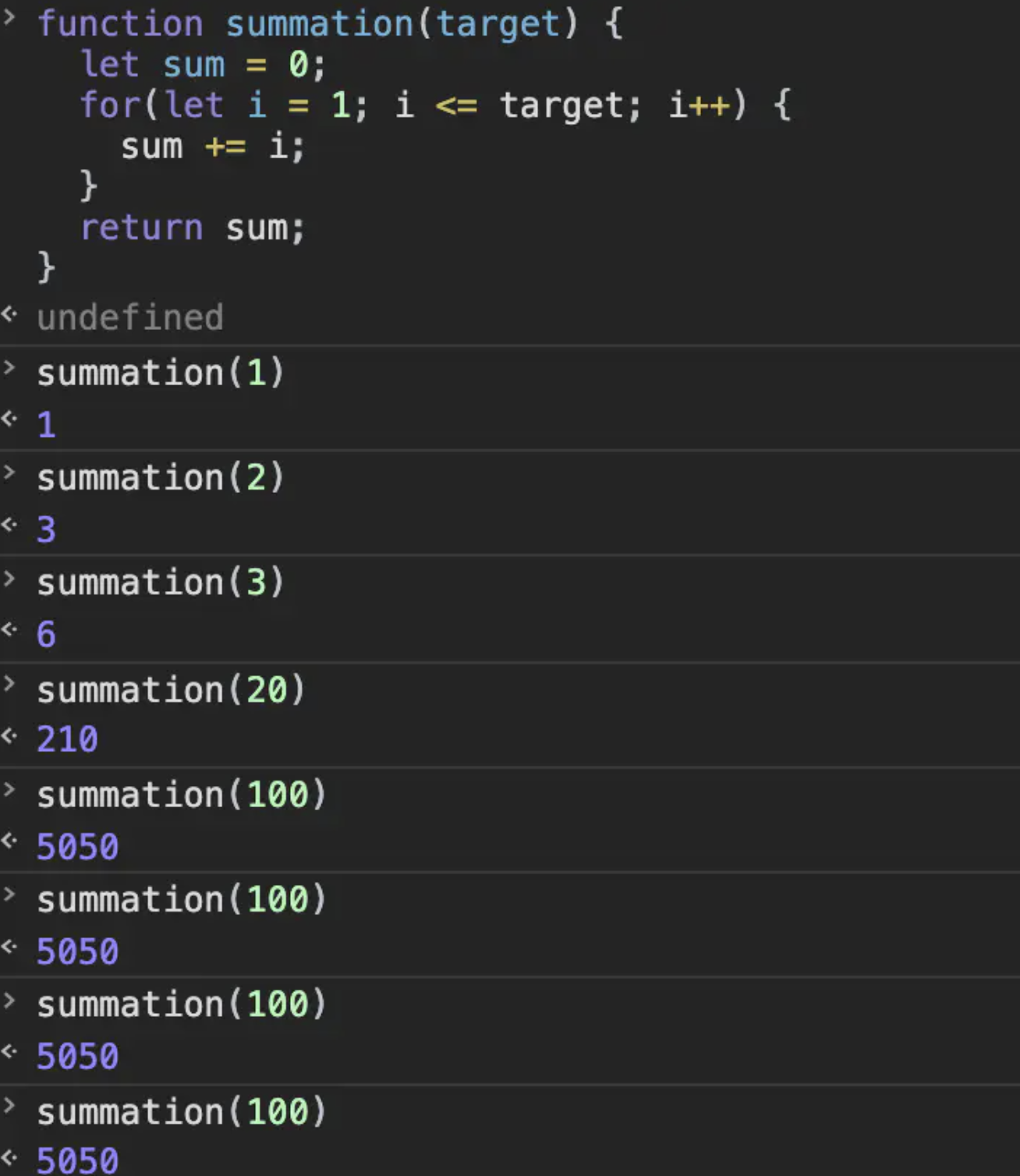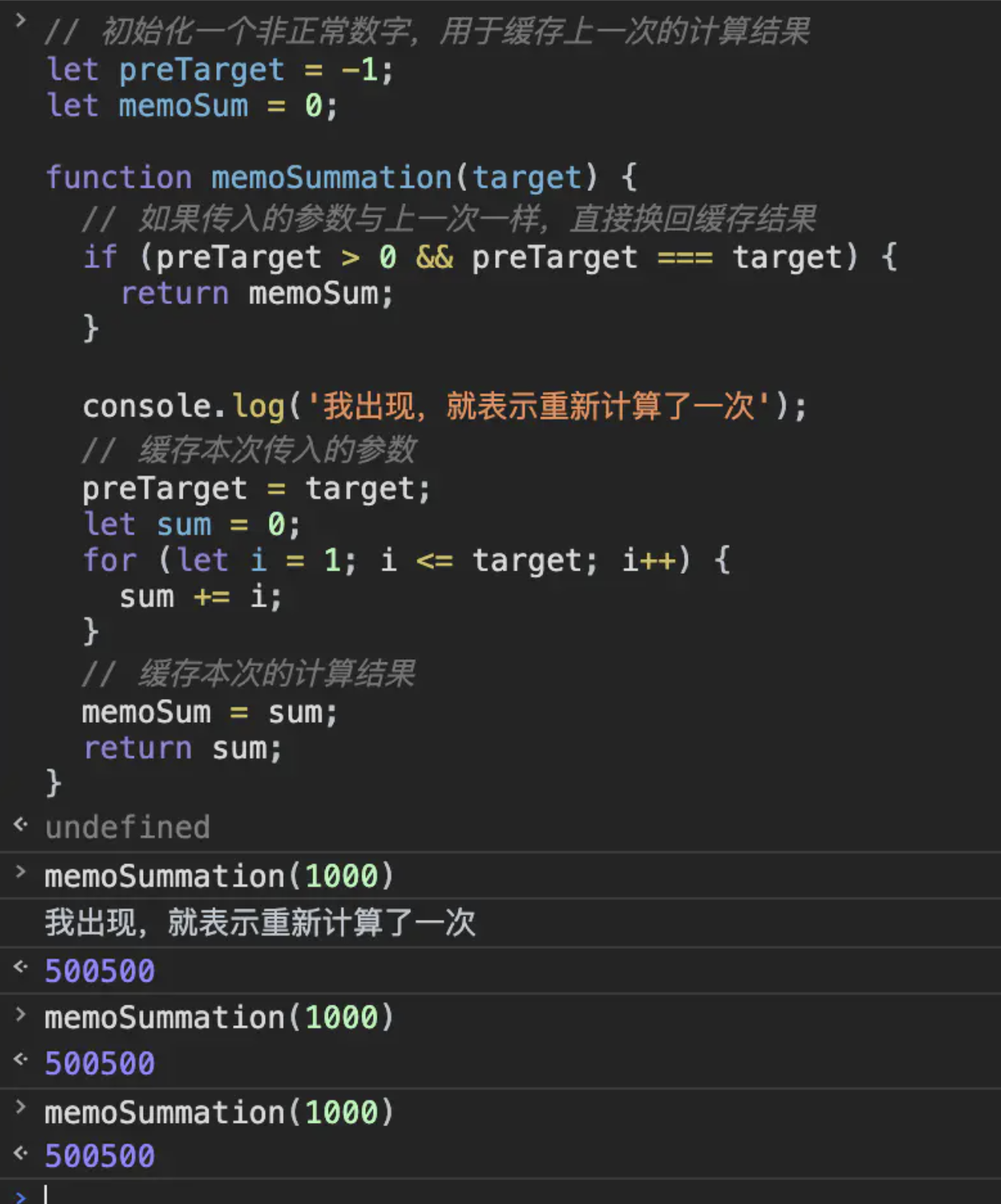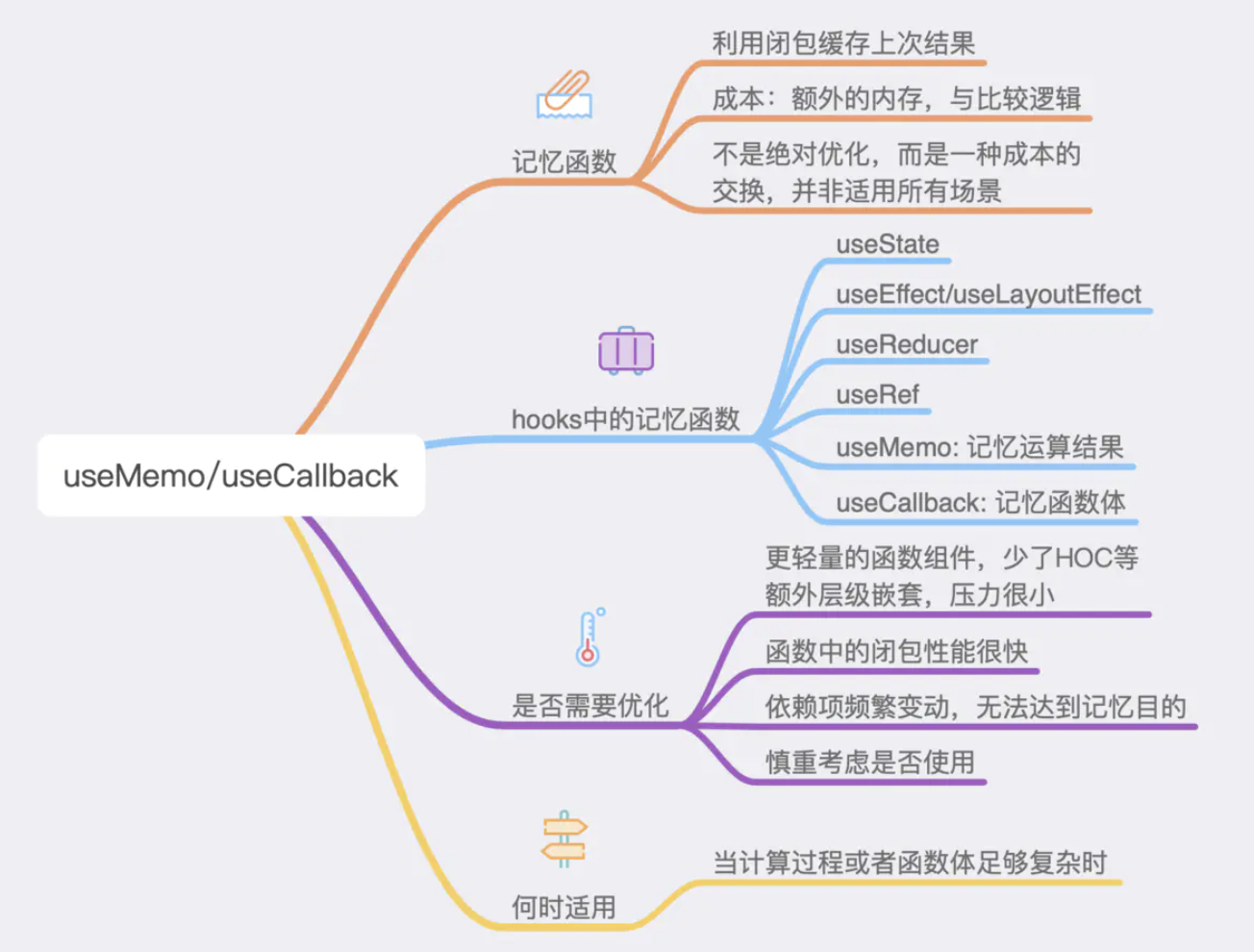``````function summation (target) {
let sum = 0
for(let i = 1; i <= sum; i++) {
sum += i
}
return sum
}
````````````// 初始化⼀个⾮正常数字，⽤于缓存上⼀次的计算结果
let preTarget = -1;
let memoSum = 0;
export function memoSummation(target: number) {
// 如果传⼊的参数与上⼀次⼀样，直接换回缓存结果
if (preTarget > 0 && preTarget === target) {
return memoSum;
}
console.log('我出现，就表示重新计算了⼀次');
// 缓存本次传⼊的参数
preTarget = target;
let sum = 0;
for (let i = 1; i <= target; i++) {
sum += i;
}
// 缓存本次的计算结果
memoSum = sum;
return sum;
}
``````1. react hooks提供的api，大多都有记忆功能。例如
useState
useEffect
useLayoutEffect
useReducer
useRef
useMemo 记忆计算结果
useCallback 记忆函数体

其他⼏个api的使⽤⽅法，我们在前⾯已经⼀⼀跟⼤家分析过。这⾥主要关注useMemo与
useCallback。

useMemo
useMemo缓存计算结果。它接收两个参数，第一个参数为计算过程(回调函数，必须返回一个结果)，第二个参数是依赖项(数组)，当依赖项中某一个发生变化，结果将会重新计算。

``````// allow undefined, but don't make it optional as that is very likely a mistake
function useMemo<T>(factory: () => T, deps: DependencyList | undefined): T;
``````

useCallback
useCallback的使用几乎与useMemo一样，不过useCallback缓存的是一个函数体，当依赖项中的一项发现变化，函数体会重新创建。

``````1 function useCallback<T extends (...args: any[]) => any>(callback: T, deps: DependencyList): T;
``````

``````import React, { useMemo, useState, useCallback } from 'react';
import { Button } from 'antd-mobile';

export default function App() {
const [target, setTarget] = useState(0);
const [other, setOther] = useState(0)

const sum = useMemo(() => {
console.log('重新计算⼀次');
let _sum = 0;
for (let i = 1; i <= target; i++) {
_sum += i;
}
return _sum;
}, [target]);

const inputChange = useCallback((e) => {
console.log(e.target.value);
}, []);

return (
<div style={{ width: '200px', margin: 'auto' }}>
<input type="text" onChange={inputChange} />
<div style={{ width: '80px', margin: '100px auto', fontSize: '40px' }}>{target} {sum}</div>
<Button onClick={() => setTarget(target + 1)}>递增</Button>
<Button onClick={() => setTarget(target - 1)}>递减</Button>
<div style={{ width: '80px', margin: '100px auto', fontSize: '20px' }}>⼲扰项 {other}</div>
<Button onClick={() => setOther(other + 1)}>递增</Button>
</div>
)
}
``````

useMemo/useCallback 的使⽤⾮常简单，不过我们需要思考⼀个问题，使⽤他们⼀定能够达到优化

React的学习经常容易陷⼊过度优化的误区。⼀些⼈在得知 shouldComponentUpdate 能够优化性

⽤更多的内存，⽤以解决计算上的冗余。
useMemo/useCallback 也是⼀样，这是⼀种成本上的交换。那么我们在使⽤时，就必须要思考，这

``````<input type="text" onChange={inputChange} />

const inputChange = useCallback((e) => {
setValue(e.target.value);
}, []);
``````

useCallback/useMemo。